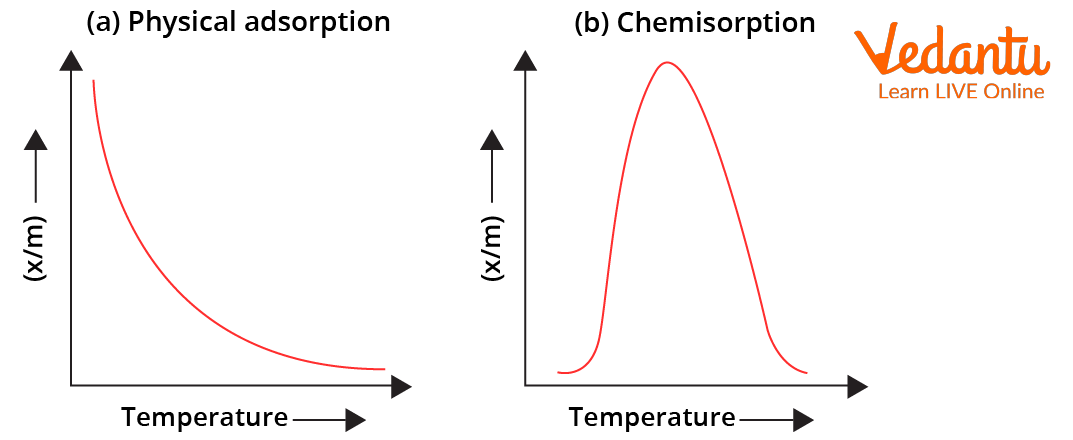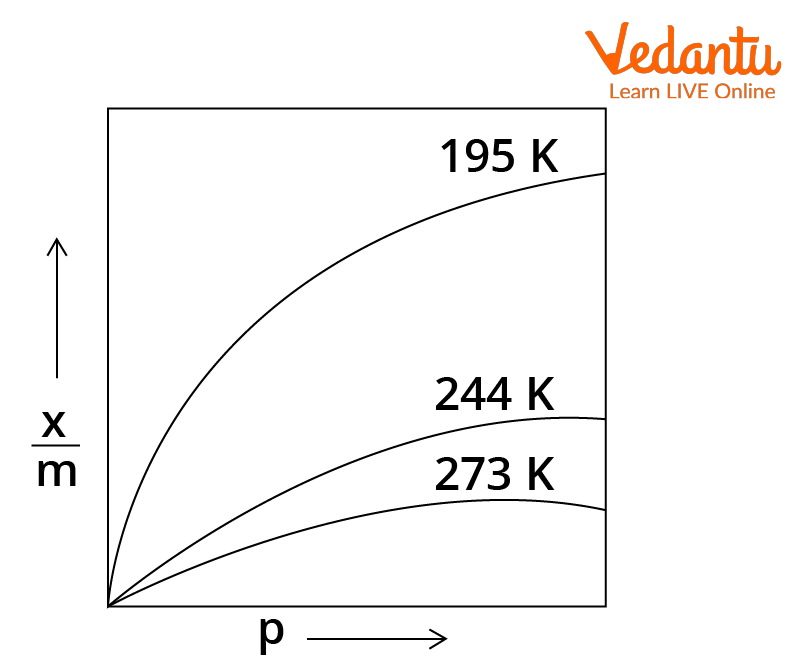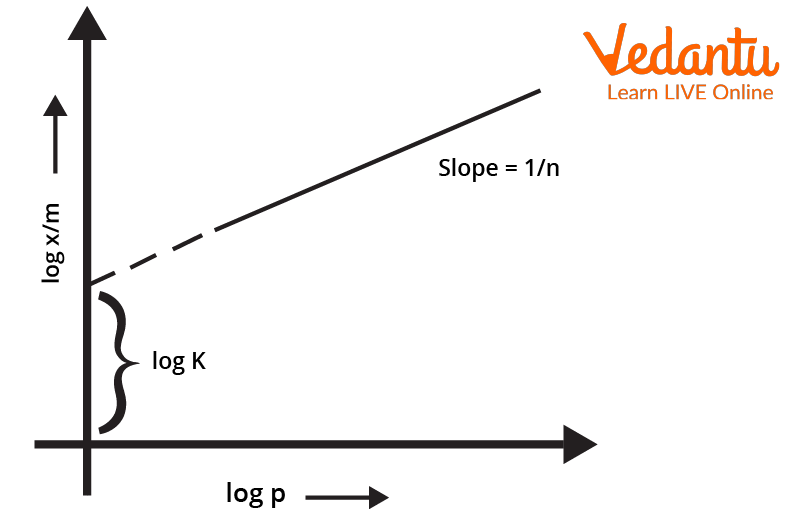Courses
Courses for Kids
Free study material
Free LIVE classes
More

# IsothermLIVE
Join Vedantu’s FREE Mastercalss

## Basis of Isotherm - Adsorption

The basis of isotherms is a process called adsorption. When the molecular species accumulate at the surface of a material rather than in its bulk, the process is known as adsorption. It is essentially a surface phenomenon where the accumulating species is called adsorbate and the material on which adsorption takes place is called adsorbent. The adsorbate is usually a gas and the adsorbent, a solid surface.

## Factors Affecting Rate of Adsorption

Adsorption is of two types- physical (physisorption) and chemical (chemisorption). Physisorption occurs on account of formation of weak Vander Waal forces. However, if chemical bond formation takes place it is termed as chemisorption. There are multiple factors that affect the rate of adsorption. Some increase the rate while others may retard it. A factor may affect different types of adsorptions differently. Effect of a factor can be studied by changing one factor and keeping others constant.

## Nature of Gas

Higher is the liquefaction tendency of gas, higher is its adsorption tendency. Liquefaction tendency of a gas can be assumed by considering attractive forces. Higher the attractive forces between gas particles, more is their liquefaction tendency and therefore, more is their adsorption.

## Nature of Solid

This factor completely depends on the surface area of the solid. More be the surface area of solid, more be the extent of adsorption.

For physisorption, the order of adsorption according to surface area is Solid piece < Powdered solid < Sol of solid

For chemisorption, d and f block elements have more adsorption tendency due to vacant valencies.

## Temperature

For physisorption, adsorption tendency decreases with increase in temperature due to decrease in attractive forces for chemisorption, adsorption tendency initially increases up to a certain extent (due to increase in fraction of molecules possessing activation energy) and then decreases.Variation of Extent of Adsorption $(\frac{x}{m})$ with Temperature

## Pressure

Adsorption of gases results in decrease in volume of the system. Therefore, it is expected that at a given temperature, the extent of adsorption will increase with increase in pressure of gas. The curves which enable us to understand and derive a mathematical relation between extent of adsorption and pressure, at a constant temperature, are called isotherms.Variation of Extent of Adsorption $(\frac{x}{m})$ with Pressure (isotherm)

## Isotherms

Since adsorption depends on various factors like temperature, pressure, surface area, etc., it is difficult to understand the dependency relation of adsorption tendency and all factors together. Therefore, all factors are kept constant while one is changed to study the effect on adsorption. When temperature is kept constant while altering the pressure, a graph called isotherm is plotted.

As seen from the isotherm the relation between pressure and adsorption is as follows:

At low pressure: Adsorption is directly proportional to pressure

At high pressure: Adsorption is independent to pressure (reaches saturation)

In between the above pressure conditions: Adsorption varies with $P^{x}$ where, $x<1$

To derive a mathematical relation from the above graph, attempts were made by two different scientists- Herbert Freundlich and Irving Langmuir.

## Freundlich Isotherm

In 1909, Freundlich gave an empirical relationship between the quantity of gas adsorbed by unit mass of solid adsorbent and pressure at a particular temperature. The relationship can be expressed by the following equation:

$\frac{x}{m} \ = \ k.P^{\frac{1}{n}}$

Where, $\frac{x}{m} \ =$ extent of adsorption (x is mass of adsorbate and m is mass of adsorbent when dynamic equilibrium is achieved)

K and n = constants that depend on adsorbent and adsorbate at particular temperatures (value of $\frac{1}{n}$ lies between $0.1 \ to \ 0.5$)

$P \ =$ pressure

## Plotting the Isotherm

To plot the freundlich isotherm, the log of the equation $\frac{x}{m} \ = \ k.P^{\frac{1}{n}}$ is considered. The equation thus becomes $log \frac{x}{m} \ = \ logk \ + \ \frac{1}{n}logP$

The validity of Freundlich isotherm can be verified by plotting the graph according to the above equation. If it turns out to be a straight line, the isotherm is valid otherwise not.Log Graph of Freundlich Isotherm

## Credibility of the Freundlich Isotherm

Freundlich isotherm explains the behaviour of adsorption in an approximate manner in an approximate manner. The value of $\frac{1}{n}$ lies between 0 and 1 (probable range $0.1 \ to \ 0.5$). Thus, the Freundlich isotherm equation holds well over a limited range of pressure

When $\frac{1}{n} \ = \ 0, \ \frac{x}{m} \ =$ constant, the adsorption is independent of pressure (Case at very high pressures)

When $\frac{1}{n} \ = \ 1, \ \frac{x}{m} \ = \ k.P,$ the adsorption varies directly with pressure (Case at very low pressures)

Experimentally, both the conditions are observed. Therefore, although Freundlich isotherm can explain the adsorption tendency with variation in pressure at certain points, it does not justify the entire graph.

Since gases approach saturation at very high pressure, Freundlich isotherms fails at high pressure as $\frac{x}{m} \ = \ k.P^{\frac{1}{n}}$ is not justified at high pressure.

## Langmuir Isotherm

It is a more widely accepted isotherm. It assumes that the adsorbed gas is ideal and only a monolayer is formed by it. Therefore, it is more accurately valid for chemisorption. Here, all adsorption sites are considered equivalent and the rate of adsorption is proportional to vacant sites. It is also assumed that adsorption is reversible and at equilibrium, rate of adsorption and desorption are equal.

## Langmuir Equation and Credibility

According to Langmuir, the equation for plotting an adsorption isotherm is $\frac{x}{m} \ = \frac{a.P}{1 \ + \ bP}$

Where, $\frac{x}{m} \ =$ extent of adsorption (x is mass of adsorbate and m is mass of adsorbent when dynamic equilibrium is achieved)

a and b = Langmuir constants

P = pressure

At very high pressure, $1 \ + \ bP \approx \ b.P$ , therefore, according to above equation- $\frac{x}{m} \ = \frac{a.P}{b.P} \ = \ \frac{a}{b}$

At very low pressure, $1 \ + \ bP \approx \ 1$ , therefore, according to above equation- $\frac{x}{m} \ = \ a.P$

Therefore, Langmuir isotherm is valid for high as well as low pressures.

## Conclusion

Adsorption, the process of accumulation of an adsorbate (gas) on an adsorbent (solid surface) is of two types- chemisorption and physisorption. Multiple factors like surface area, temperature, pressure, etc. affect adsorption. At constant temperature, a graph depicting the variation of adsorption with change in pressure is called an isotherm. Two attempts were made to derive equations for adsorption. The Freundlich isotherm equation is represented by $\frac{x}{m} \ = \ k.P^{\frac{1}{n}}$ . Only if the log of this equation is a straight line, the freundlich isotherm is valid. It fails at high pressure. The Langmuir isotherm equation is depicted by $\frac{x}{m} \ = \frac{a.P}{1 \ + \ bP}$ . It is more credible since it is valid at high as well as low pressure conditions.

Last updated date: 26th Sep 2023
Total views: 112.2k
Views today: 2.12k

## FAQs on Isotherm

1. How does adsorption take place?

A molecule at the surface has unbalanced force due to exposure to another surface from one side. In order to satisfy the unbalanced forces, the molecules at the surface attract and retain other molecular species, when the substance is brought in contact with them. Due to adsorption, there is always a decrease in residual forces and thus $\Delta H_{(adsorption)}$ is negative but $\Delta S_{(adsorption)}$ is also negative. Therefore, adsorption is favoured at temperature conditions when $|\Delta H| \ > \ T|\Delta S|$

2. What is the relation of Freundlich isotherm and its drawbacks?

Freundlich isotherm follows the relation $\frac{x}{m} \ = \ k.P^{\frac{1}{n}}$

Where, $\frac{x}{m} \ =$ extent of adsorption (x is mass of adsorbate and m is mass of adsorbent when dynamic equilibrium is achieved)

k and n = constants that depend on adsorbent and adsorbate at particular temperatures (value of $\frac{1}{n}$

lies between 0.1  to  0.5)

P = Pressure

It holds to be true at low pressures but at high pressures, when adsorption reaches saturation, it tends to fail.

3. What are three assumptions in Langmuir isotherm?

Langmuir isotherm is more accurate than Freundlich isotherm. However, it is based on several assumptions. The first assumption is that the adsorbed gas is ideal. The second assumption states that only a monolayer is formed by the adsorbed gas, making Langmuir isotherm more appropriate for chemisorption. The next assumption states that every adsorption site is equivalent and that the rate of adsorption depends directly upon the fraction of vacant sites at surface. Lastly, it assumes that adsorption is reversible and at equilibrium the rate of adsorption is equal to the rate of desorption.

The equation for Langmuir isotherm is $\frac{x}{m} \ = \frac{a.P}{1 \ + \ bP}$.